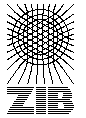# Testset P4E

These instances are random generated complete graphs with euclidian weights. The were introduced in CGR92 .

Name   |V|    |E|    |T|    DC    Opt
P455100 4950 Ps 1138
P456100 4950 Ps 1228
P457100 4950 10 Ps 1609
P458100 4950 10 Ps 1868
P459100 4950 20 Ps 2345
P460100 4950 20 Ps 2959
P461100 4950 50 Ps 4474
P463200 19900 10 Ps 1510
P464200 19900 20 Ps 2545
P465200 19900 40 Ps 3853
P466200 19900 100 Ps 6234

The column DC classifies the difficulty of the instance.

L
Solvable by usage of local preprocessing. Typical examples are the SD-Test, BD-n Tests and FST computations. Neither a global upper nor lower bound needs to be computed.
P
Solvable by polynomial time algorithms, like dual ascent in combination with primal heuristic, a integral LP formulation or advanced preprocessing like reduced cost criteria or the RCR-Test.
NP
No polynomial time algorithm is known. Use of an exponential time enumeration sceme like Branch-and-Bound is neccessary.

The letter after class gives an impression how long it takes to solve the problem using state-of-the-art soft- and hardware. secounds means less than a minute (this includes instances which can be solved in fractions of a second). minutes means less than an hour. hours is less than a day and days is less than a week. weeks mean it takes really a long time to solve this instance. ? means the instance is not solved or the time is not known.

If the number in the Opt column is written in italics the optimum is not known. The number given is the best know upper bound.

Last Update : 2015/02/11 11:57:20 \$ by Thorsten Koch